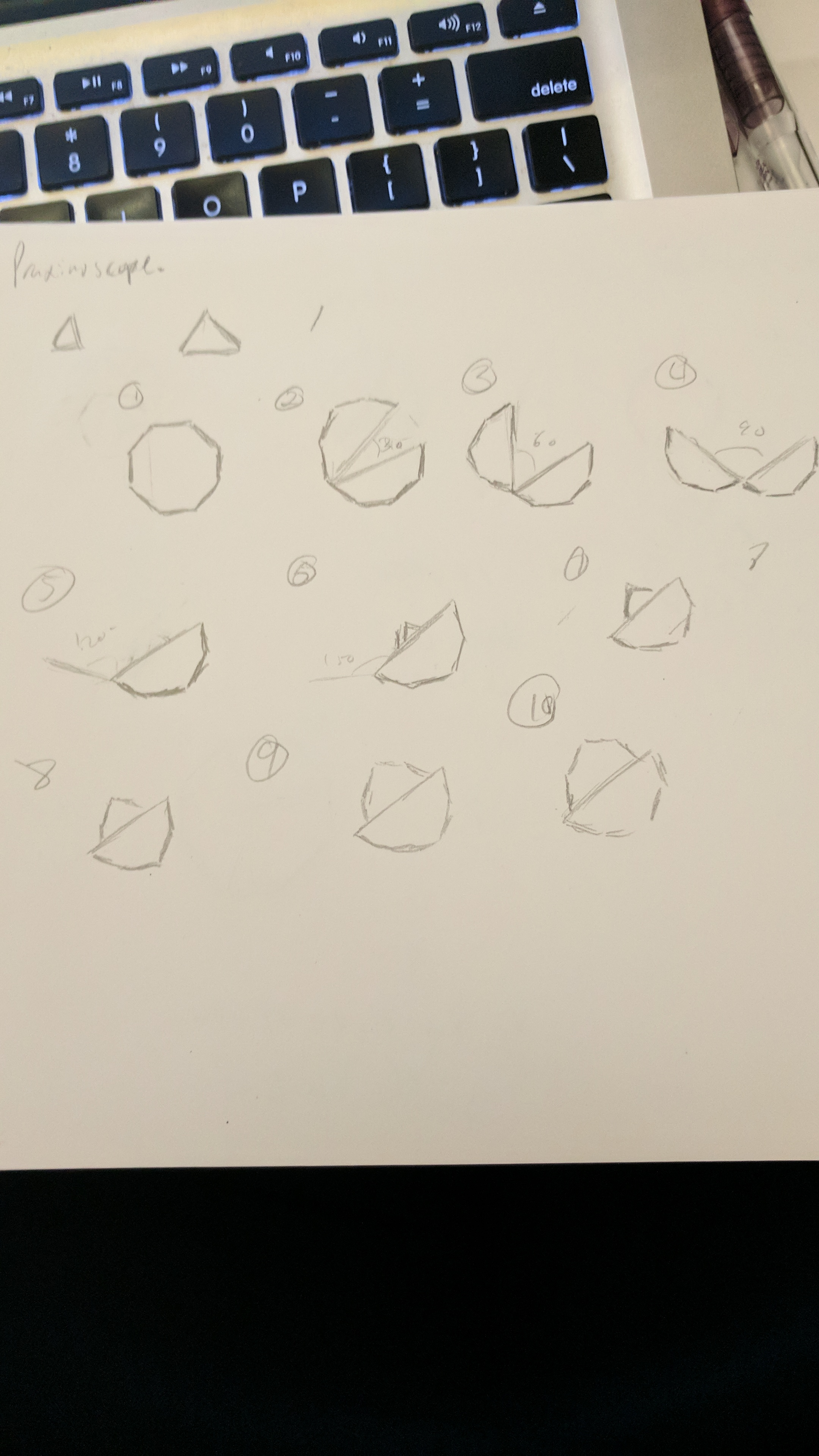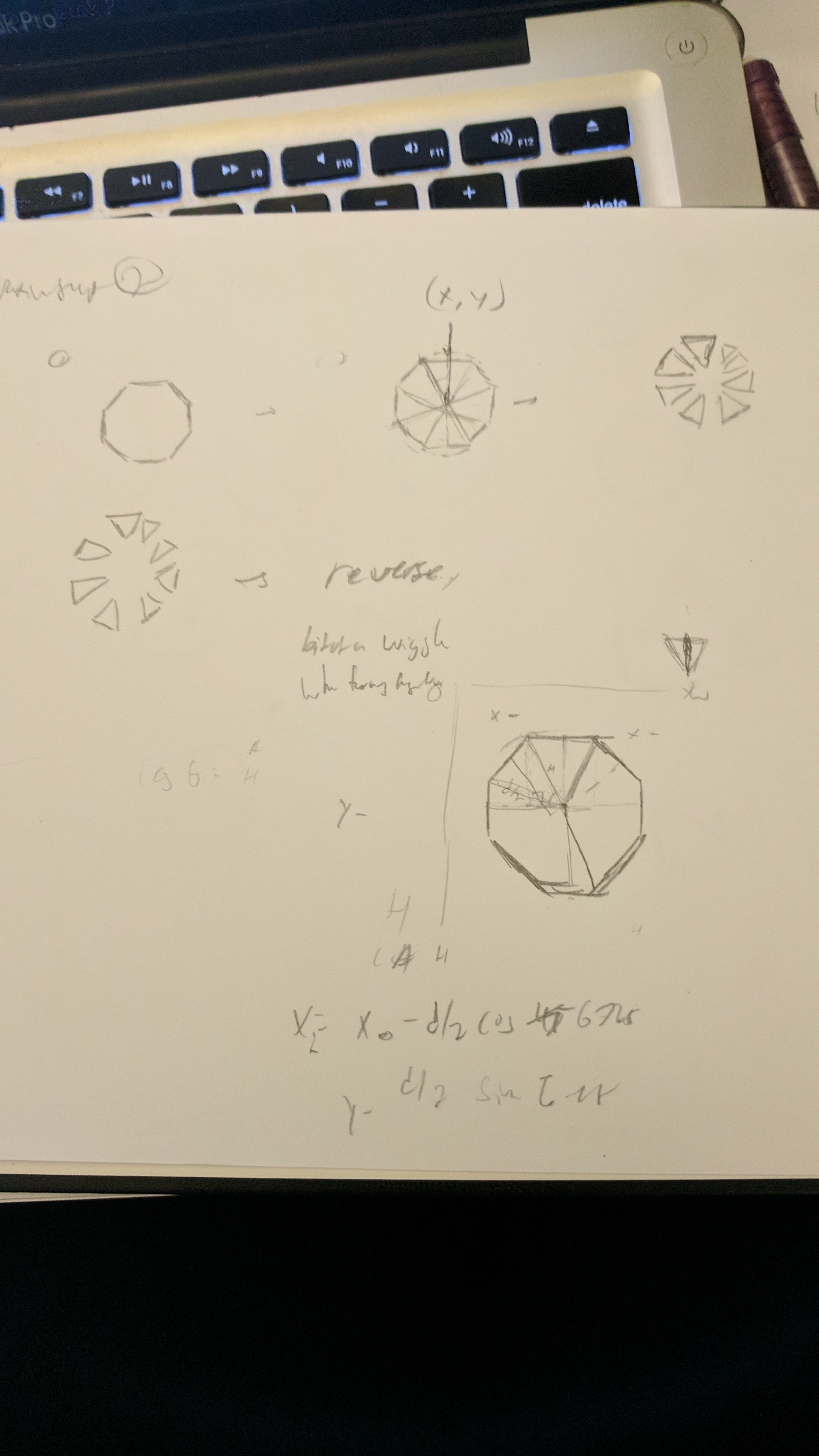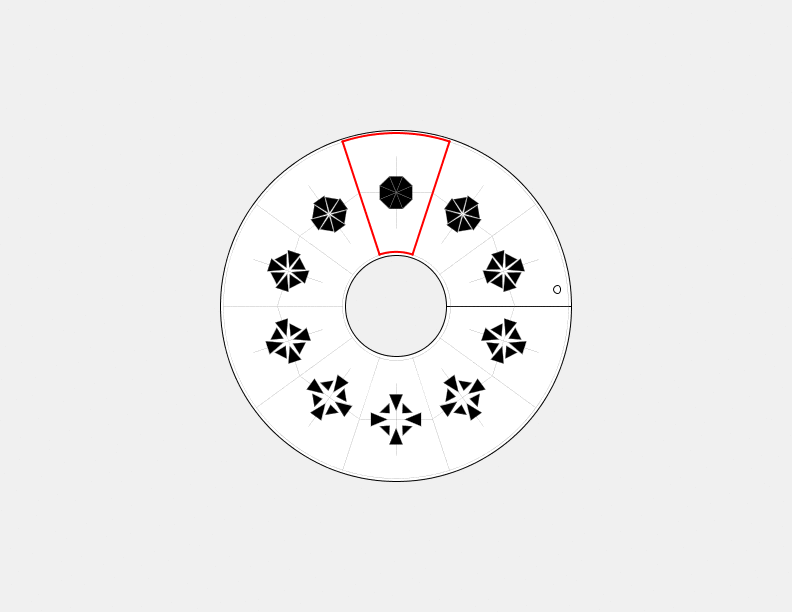# aahdee-Scope

Below is my initial design.I really like this design, but this seemed like a rather large project for something that is due tomorrow, so I decided to save it for a rainy day. Next is my simplified design.As seen above, I was going to have all of the triangles pop out. When writing this program, I first made all of the inner points move in and out and then went on to do the outer points. But when I went to make the diagonal triangles move, I liked the current pulsating animation so much that I kept it as it is. Finally, the final design.Here is a pdf:
praxinoscope-output

Below is my code:

```float diameter = 44; float ex = 0;   void octagon(float ex, int x, int y, float diameter) { float angle1 = 45; float angle23 = 67.5;   //points float nx2 = x-((diameter/2)*cos(radians(angle23))), ny2 = y-((diameter/2)*sin(radians(angle23))); float nx3 = x+((diameter/2)*cos(radians(angle23))), ny3 = y-((diameter/2)*sin(radians(angle23))); float sx2 = x-((diameter/2)*cos(radians(angle23))), sy2 = y+((diameter/2)*sin(radians(angle23))); float sx3 = x+((diameter/2)*cos(radians(angle23))), sy3 = y+((diameter/2)*sin(radians(angle23))); float ex2 = x+((diameter/2)*cos(radians(angle1/2))), ey2 = y-((diameter/2)*sin(radians(angle1/2))); float ex3 = x+((diameter/2)*cos(radians(angle1/2))), ey3 = y+((diameter/2)*sin(radians(angle1/2))); float wx2 = x-((diameter/2)*cos(radians(angle1/2))), wy2 = y-((diameter/2)*sin(radians(angle1/2))); float wx3 = x-((diameter/2)*cos(radians(angle1/2))), wy3 = y+((diameter/2)*sin(radians(angle1/2))); //N triangle(x, y-ex, nx2, ny2-ex, nx3, ny3-ex); //S triangle(x, y+ex, sx2, sy2+ex, sx3, sy3+ex); //E triangle(x+ex, y, ex2+ex, ey2, ex3+ex, ey3); //W triangle(x-ex, y, wx2-ex, wy2, wx3-ex, wy3); //NE triangle(x+(ex*cos(radians(45))), y-(ex*sin(radians(45))), nx3, ny3, ex2, ey2); //NW triangle(x-(ex*cos(radians(45))), y-(ex*sin(radians(45))), nx2, ny2, wx2, wy2); //SW triangle(x-(ex*cos(radians(45))), y+(ex*sin(radians(45))), wx3, wy3, sx2, sy2); //SE triangle(x+(ex*cos(radians(45))), y+(ex*sin(radians(45))), sx3, sy3, ex3, ey3); }   //------------------------------------------------------- void drawArtFrame (int whichFrame) { fill(0); if (whichFrame < nFrames/2){ ex = map(whichFrame, 0, nFrames/2, 0, diameter/4); }   if (whichFrame >= nFrames/2){ ex = diameter/4-map(whichFrame, nFrames/2, nFrames, 0, diameter/4); } octagon(ex, 0, 0, diameter); }```